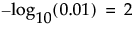• Standard Least Squares
 • Nominal Logistic
 • Ordinal Logistic
 • Proportional Hazard
 • Parametric Survival
 • Generalized Linear Model
Effect Summary Report shows the initial view of the Effect Summary report for the Fitness.jmp data table. The check box labeled FDR controls the columns that appear in the summary table.
Effect Summary Report
Shows the LogWorth for each model effect, defined as -log10(p-value). This transformation adjusts p-values to provide an appropriate scale for graphing. A value that exceeds 2 is significant at the 0.01 level (because).
Shows the False Discovery Rate LogWorth for each model effect, defined as -log10(FDR PValue). This is the best statistic for plotting and assessing significance. Select the FDR check box to replace the LogWorth column with the FDR LogWorth column.
Shows the p-value for each model effect. This is generally the p-value corresponding to the significance test displayed in the Effect Tests table or Effect Likelihood Ratio Tests table of the model report.
Shows the False Discovery Rate p-value for each model effect calculated using the Benjamini-Hochberg technique. This technique adjusts the p-values to control the false discovery rate for multiple tests. Select the FDR check box to replace the PValue column with the FDR PValue column.
Opens a panel that contains a list of all columns in the data table. Select columns that you want to add to the model, and then click Add below the column selection list to add the columns to the model. Click Close to close the panel. Effect Summary Add Columns Panel shows the Add Columns panel.